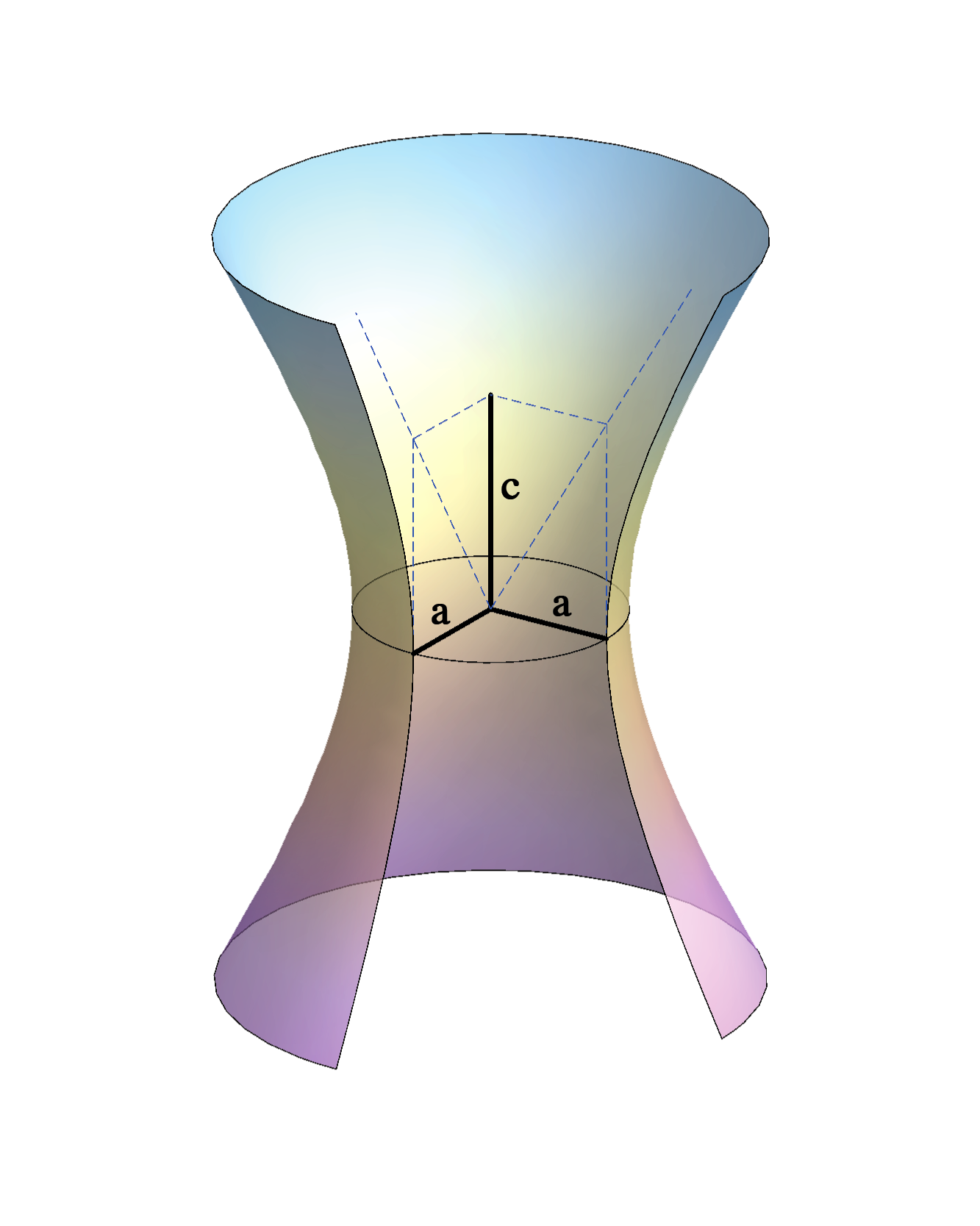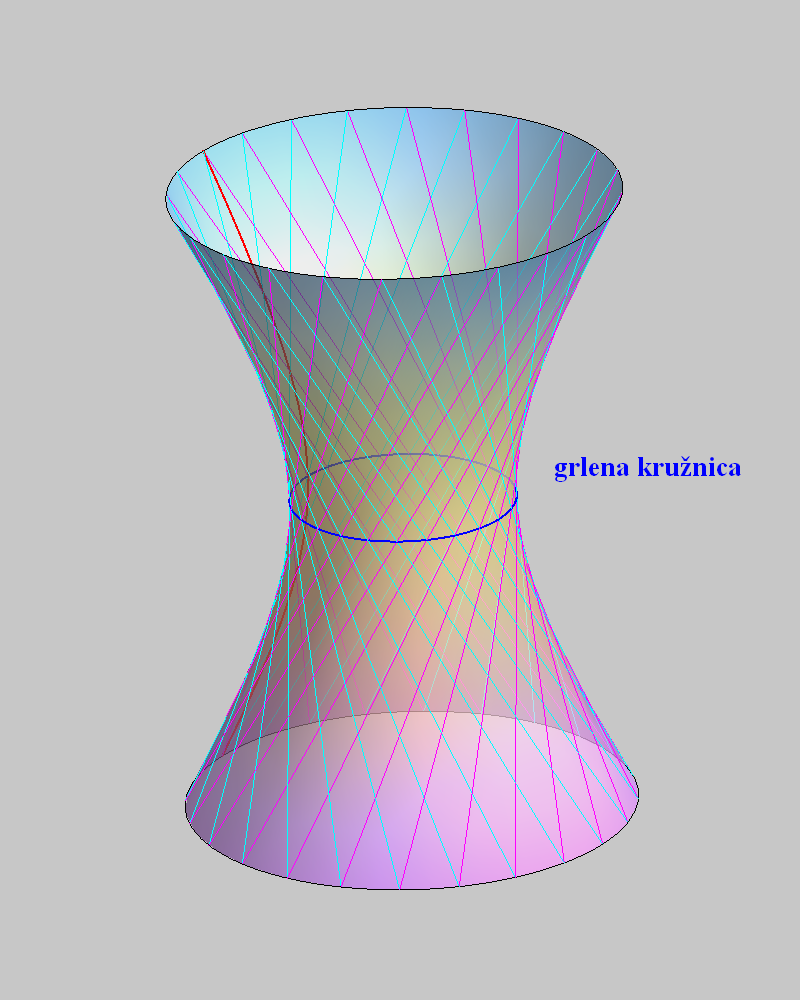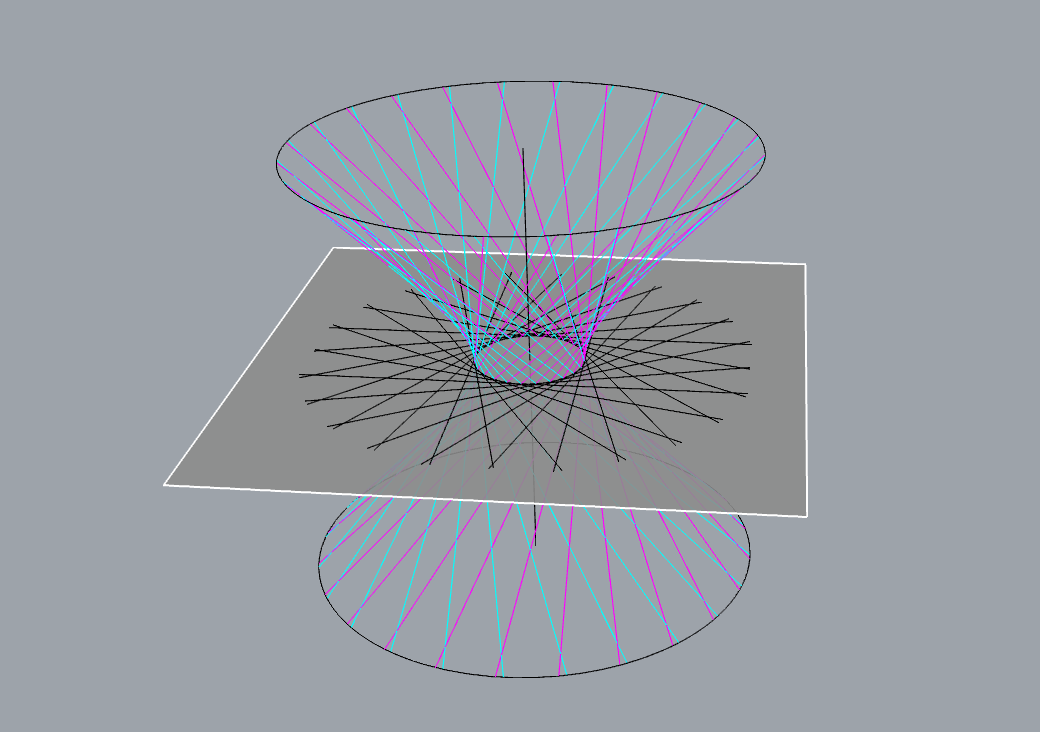## Circular hyperboloid of one sheet

$$\frac{x^2}{a^2}+\frac{y^2}{a^2}-\frac{z^2}{c^2}=1$$

Obtained by rotating a hyperbola around its conjugate (minor) axis,right-click $$\rightarrow$$ PLAY

or

by rotating one line around another line when these lines are skew.

right-click $$\rightarrow$$ PLAY

This hyperboloid is doubly ruled surface - has two systems of ruling.

• Through each point passes one ruling from each system.

• All rulings in one system are skew lines.

• Each ruling from one system intersects all rulings from the other system.• On the smallest circle of the hyperboloid lie the vertices of cross-section hyperbolas(intersections with planes that contain the axis).

• Smallest circle contains those points of the rulings that are closest to the axis.

• Orthogonal projections of the rulings to the plane of the smallest circle (or a plane parallel to that plane) are tangents of the smallest circle.

• Tangent planes in the points on the smallest circle are parallel to the axis.### Asymptotic cone of one-sheeted hyperboloid

right-click $$\rightarrow$$ PLAY

Created by Sonja Gorjanc, translated by Helena Halas and Iva Kodrnja - 3DGeomTeh - Developing project of the University of Zagreb